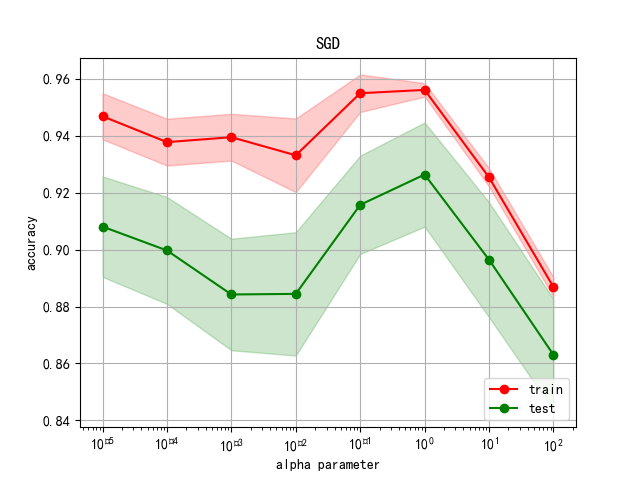## 介绍

Scikit-learn提供了learning_curve类，方便获得和训练的可视化相关的数据。

Python机器学习可视化（一）sklearn.learning_curve``````from sklearn.learning_curve import learning_curve, validation_curve
``````

``````from sklearn.datasets import load_digits
X = digits['data'] #(1797,64)
Y = digits['target'] #(1797,)
``````

2.采用SGD分类器

``````from sklearn.linear_model import SGDClassifier
sgd = SGDClassifier(loss='log', shuffle=True, n_iter=5, penalty='l2', alpha=0.0001, random_state=3)

``````

3.选择超参数区间：10的-2次方到10的5次方，共8个值

``````testing_range = np.logspace(-5,2,8)  #自变量
``````

## 调用validation_curve函数

``````train_scores, test_scores = validation_curve(sgd, X, Y, param_name='alpha',param_range=testing_range,
cv=10, scoring='accuracy', n_jobs=1)
``````

1.输入
sgd:分类器
X: data
Y: 标签
cv: K折交叉验证，本文选择10
scoring: sklearn一般评估方法

param_name: 需要调整的超参数名称
param_range:超参数区间
2.输出：
train_scores:对于8个超参数的训练集，对10折交叉验证的10个训练得分，本文为(8,10)
test_scores:对于8个超参数的测试集，对10折交叉验证的10个测试得分，本文为(8,10)

## 处理

1.对于8种不同参数的训练集，对10折交叉验证的10个训练/测试得分取平均值(即压缩列)。
2.得到得分范围的上下界
3.如果上下界区域太大，可以在np.std前乘以一个小于一的数

``````mean_train = np.mean(train_scores,1)  #(8,)
# 得到得分范围的上下界
upper_train = np.clip(mean_train + np.std(train_scores,1),0,1)
lower_train = np.clip(mean_train - np.std(train_scores,1),0,1)

mean_test = np.mean(test_scores,1)
# 得到得分范围的上下界
upper_test = np.clip(mean_test + np.std(test_scores,1),0,1)
lower_test = np.clip(mean_test - np.std(test_scores,1),0,1)
``````

## 画图

``````plt.figure('Fig2')
plt.semilogx(testing_range,mean_train,'ro-',label='train')
plt.plot(testing_range,mean_test,'go-',label='test')
##填充上下界的范围
plt.fill_between(testing_range,upper_train,lower_train,alpha=0.2,#alpha：覆盖区域的透明度[0,1],其值越大，表示越不透明
color='r')
plt.fill_between(testing_range,upper_test,lower_test,alpha=0.2,#alpha：覆盖区域的透明度[0,1],其值越大，表示越不透明
color='g')
plt.grid()
plt.xlabel('alpha parameter')
plt.ylabel('accuracy')
plt.legend(loc='lower right')
plt.title('SGD')
plt.savefig('alpha_parameter.png')
plt.show()

``````

《数据科学导论——Python语言实现》

### 【kubernetes/k8s概念】kube-ovn-controller 源码分析之一_张忠琳的博客-程序员秘密

kube-ovn-controller 逻辑职责是翻译 kubernetes 网络概念到 OVN，可以理解为 kube-ovn 的控制面功能，通过 kube-apiserver watch 所有网络相关事件，包括 Pod creation/deletion, Service/Endpoint modification, Networkpolicy changes 等等。然后翻译转为 OVN 逻辑网络的修改。也 watch kube-ovn 添加的 CRD，比如 VPC Subnet Vlan IP ...

### ES6 核心特性_前端大全的博客-程序员秘密

（给前端大全加星标，提升前端技能）作者：浪里行舟https://github.com/ljianshu/Blog/issues/10前言ES6 虽提供了许多新特性，但我们...

### HBase vs. BigTable Comparison - HBase对比BigTable_技术笔记本的博客-程序员秘密

HBase is an open-source implementation of the GoogleBigTable architecture. That part is fairly easy to understand and grasp. What I personally feel is a bit more difficult is to understand how muc

### Windows Server 2008 R2 搭建网站教程--自学笔记_Richard_Panda的博客-程序员秘密

1.最主要的一步,首先安装Windows server2008r2(现在网上流传的激活码基本都用不了了,所以就按着30天试用期来搭建网站,熟悉一下简要流程)2.打开菜单栏中的"服务管理器",然后点击里面的"添加角色"3.首先安装web服务器(IIS),选择后,按下一步4.继续下一步5.勾选：应用程序开发、日志、跟踪、性能全选、IIS6兼容性、FTP，然后下一步。如果不确定以后会用到哪些...